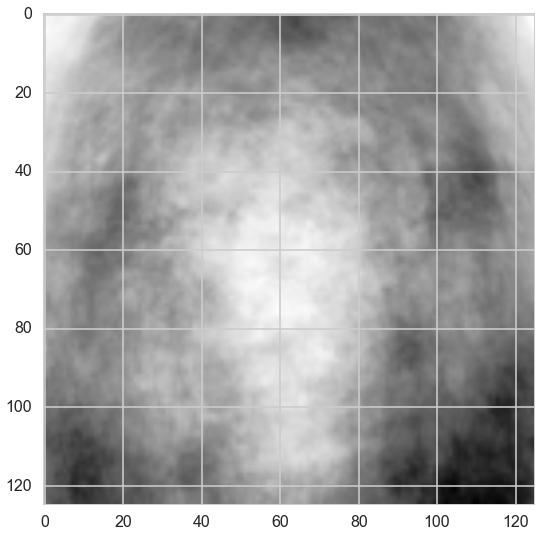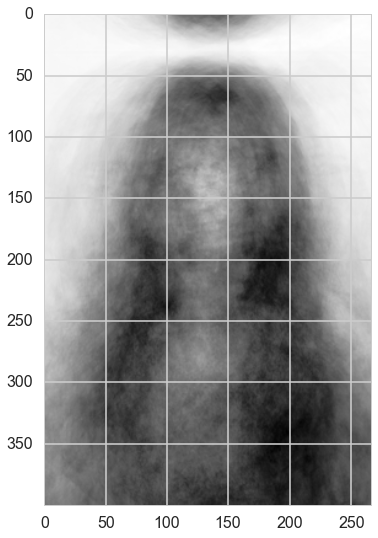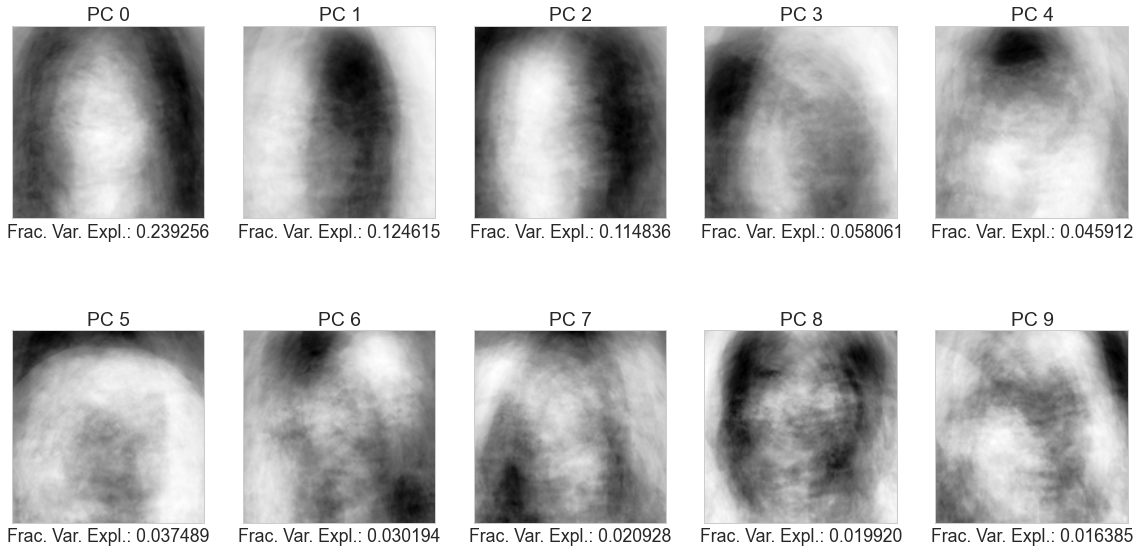# The Bachelor: Image Processing and Analytics¶

Cusworth, Heal, Leinicke, Rodriguez.

We obtain (scrape) photos of most contestants from Seasons 13-19 of The Bachelor. We register (align) the grayscale images, crop them to a small region containing only the face and face-framing hair, then perform PCA on those cropped images. We then examine the contestants' varying levels of success as represented by the projection of the images onto the first two principal components. We see that in this PC-1&2 space, the 'winners' and 'runners up' classes can be separated by a linear classifier.

Due to the high dimensionality of this data set, we would give this notebook about 2 hours to run.

# 1. Scrape Data¶

In :
%matplotlib inline
import numpy as np
import scipy as sp
import matplotlib as mpl
import matplotlib.cm as cm
import matplotlib.pyplot as plt
import pandas as pd
import time
pd.set_option('display.width', 500)
pd.set_option('display.max_columns', 100)
pd.set_option('display.notebook_repr_html', True)
import seaborn as sns
sns.set_style("whitegrid")
sns.set_context("poster")

from pyquery import PyQuery as pq
from bs4 import BeautifulSoup
import requests


This 'sirlinksalot' seemed like a really promising place to find photos. It pointed us in the direction of other good sites too!

In :
linkVec = []
for thisPage in :#[2,3,4,5]:
rows = soup.find_all("a")
for row in rows:
rowString = str(row)
checkASCII = [ord(letter) for letter in rowString]
if max(checkASCII)<127 and min(checkASCII)>9:

In :
print len(linkVec)

1753

In :
#sort our vector to group similar website roots

In :
archivedLinkVec = []
count = 0;
if soup.find_all("div", attrs={"class": "date captures"}):
row = soup.find_all("div", attrs={"class": "date captures"})
row = row.find("a").get("href")
count = count+1
#print count, " out of ", length, " done"

In :
#we can assume that each article from the Examiner website will have similar HTML formatting.
#So let us also note the root page.
rootPage = []

In :
#collect into a data frame

In :
myGroups = linkDF.groupby('root_page')

In :
count=0
if group:
count = count+1
print "There are",count, "unique groups." #this shows that the number of unique websites
#whose formatting we need to learn is pretty limited.

['blog.zap2it.com' 'insidetv.ew.com' '' 'www.digitalspy.com'
'www.eonline.com' 'www.examiner.com' 'www.people.com' 'www.usmagazine.com']
There are 7 unique groups.

In :
#SEASON 13 BLUE BACKGROUND
season13Names =[]
soup = BeautifulSoup(testPage.text, "html.parser")
scripts = soup.find_all("script")
for script in scripts:
if script.string:
if "Slideshow.SlideshowNavigator" in str(script.string):
mystring = str(script.string)
myvec = mystring.split("{")
myvec = myvec[7:32]
for k in myvec:
fromhere = str(k).find("Title")+8
tohere = str(k).find("Blurb")-3
season13Names.append(k[fromhere:tohere])
fromhere = str(k).find("ImageId")+9
tohere = str(k).find("PhotoCredit")-2

Out:
(25, 25)
In :
#### SEASON 14 BLUE BACKGROUND
season14Names = []
soup = BeautifulSoup(testPage.text, "html.parser")
rows = soup.find_all("img")
for row in rows:
if row.get("src"):
if "the-bachelor-14" in row.get("src"):
myname = str(row.get("alt"))
myname = myname.split("14 ")
season14Names.append(myname)
#season14Names = ["Alexa Mcallister","Ali Fedotowsky","Ashleigh Hunt","Ashley Almore","Caitlyn McCabe","Channy Choch","Christina McCasland","Corrie","Elizabeth Kitt","Elizabeth Kreft","Ella Nolan","Emily Harkins","Gia Alliemand","Jessie Sulidis","Kathryn","Kimberly","Kirsten","Michelle Kujawa","Rozlyn Papa","Sheila","Stephanie","Tenley","Tiana","Valishia Savage","Vienna Girardi"]

Out:
(26, 26)
In :
#SEASON 15 BLUE BACKGROUND
season15Names=[]
soup = BeautifulSoup(testPage.text, "html.parser")
rows = soup.find_all("img")
for row in rows:
if row.get("src"):
if "the-bachelor-15" in row.get("src"):
season15Names.append(str(row.get("alt")))


Out:
(28, 28)
In :
#SEASON 16 BLUE BACKGROUND
season16Names = []
soup = BeautifulSoup(testPage.text, "html.parser")
rows = soup.find_all("img")
for row in rows:
if row.get("data-lazy-src"):
if "The_Bachelor_16_" in row.get("data-lazy-src"):
season16Names.append(str(row.get("alt")))

Out:
(26, 26)
In :
#SEASON 17 BLUE BACKGROUND
season17Names = []
soup = BeautifulSoup(testPage.text, "html.parser")
rows = soup.find_all("img")
for row in rows:
if row.get("data-lazy-src"):
if "-sean-lowe-season" in row.get("data-lazy-src"):
season17Names.append(str(row.get("title")))

Out:
(25, 25)
In :
#SEASON 18 BLUE BACKGROUND
season18Links=["http://www.usmagazine.com/entertainment/pictures/the-bachelor-season-18-meet-juan-pablos-bachelorettes-2013412/343%d" % k for k in range(71,98)]
season18Names = []
soup = BeautifulSoup(testPage.text, "html.parser")
rows = soup.find_all("figure")
for row in rows:
if row.get("data-slug"):
if "18-meet-juan-pablos" in row.get("data-slug"):
thisimg = row.find_all("img")
thisimg = thisimg.get("src")
season18Names.append(str(row.get("data-title")))

Out:
(27, 27)
In :
#SEASON 19 BLUE BACKGROUND
season19Names =[]
soup = BeautifulSoup(testPage.text, "html.parser")
rows = soup.find_all("img")
for row in rows:
if row.get("alt"):
if "starring Chris Soules" in row.get("alt"):
b = row.get("alt")
season19Names.append(b.split())

Out:
(30, 30)
In :
#AGGREGATE PICS AND NAMES
allNames = np.concatenate([season13Names,season14Names,season15Names,season16Names,season17Names,season18Names,season19Names])


Out:
(187, 187)

# 2. Save links to local machine & Turn into NumPy Arrays¶

In :
import PIL
from PIL import Image


Uncomment this block if running for the first time.

In :
## SAVE ALL PICS TO LOCAL MACHINE
## only really need to do this once
## make sure you're in the right folder on your local machine

# import urllib
# count = 0
#     count = count+1


In :
picsAsMatrices = []
old_allNames = allNames
print len(old_allNames)
allNames = []
viableIndices = np.concatenate([range(0,5),range(6,11),range(12,20),range(21,25),range(26,105),range(106,187)])
for count in viableIndices:
allNames.append(old_allNames[count])

187

In :
picsAsBinaryMatrices = []
#turn pics into B&W, then into (binary) numpy arrays
for count in viableIndices:
img0 = Image.open("bachelorette_pics/bachelorette%d.jpg" % count).convert('LA')
img0.save("greyscale_pics/greyscale%d.png" % count)
img1 = np.array(img0.getdata())
img2 = (img1[:,1]-img1[:,0]).reshape(img0.size, img0.size)
picsAsBinaryMatrices.append(img2)


Test code

In :
#plt.imshow(picsAsBinaryMatrices);
#im1 = picsAsBinaryMatrices
#im2 = picsAsBinaryMatrices
#mat1 = np.array(im1.getdata())#.reshape(im1.size, im1.size, 2)
#mat11= (mat1[:,1]-mat1[:,0]).reshape(im1.size, im1.size)
#plt.imshow(mat11)

#heights,widths=[],[]
#for pic in picsAsBinaryMatrices:
#    heights.append(pic.size)
#    widths.append(pic.size)


Test code. I tried to use PIL but couldn't get eigenvalues, eigenvectors, etc. with it.

So, I made my own registration package (see next section).

In :
#cropHeight = min(heights)
#cropWidth = min(widths)
#cropParams = [0,0,cropHeight,cropWidth]

#im1 = im1.crop(cropParams)
#im2 = im2.crop(cropParams)
#Image.blend(im1,im2,alpha=0.5)

#newIm = picsAsBinaryMatrices
#newIm = newIm.crop(cropParams)
#for pic in picsAsBinaryMatrices:
#    tempPic = pic.crop(cropParams)
#    newIm = Image.blend(newIm,tempPic,alpha=0.25)

##test
#plt.imshow(newIm)


# 3. Registration¶

In :
#REGISTRATION, using cross-correlation.
from scipy import signal
from scipy import misc


Test code

In :
#decRate = 20
#sig0 = [[0,0,0],[0,1,0],[0,0,0]]
#sig1 = picsAsBinaryMatrices[85:135,125:175]
#sig2 = picsAsBinaryMatrices[80:130,100:150]
#decSig1 = sp.misc.imresize(sig1, decRate, interp='nearest', mode=None)
#decSig2 = sp.misc.imresize(sig2, decRate, interp='nearest', mode=None)
#xcorr = sp.signal.correlate2d(sig0,sig0, mode='full', boundary='symm')

#print xcorr
#print
#print xcorr.shape
#plt.imshow(xcorr);

#plt.imshow(decSig1)

#subset4= picsAsBinaryMatrices[testnum][50:(50+framesize),50:(50+framesize)]
#subset5= picsAsBinaryMatrices[refnum][50:(50+framesize),50:(50+framesize)]
#xcorr = sp.signal.correlate2d(picsAsBinaryMatrices[refnum],subset4, mode='same', boundary='symm')
#print (picsAsBinaryMatrices[refnum]).shape

#decimated = sp.misc.imresize(picsAsBinaryMatrices, 10, interp='bilinear', mode=None)
#plt.imshow(decimated)

In :
refnum = 6
testnum = 17
framesize = 150

In :
#mirror image borders
def mirror_image(im):
im2 = np.concatenate((im[:,::-1],im),axis=1)
im2 = np.concatenate((im2,im[:,::-1]),axis=1)
mirrim = np.concatenate((im2,im2[::-1,:]),axis=0)
mirrim = np.concatenate((im2[::-1,:],mirrim),axis=0)
return mirrim

In :
#find index of max cross-correlation
#from http://stackoverflow.com/questions/21989513/finding-index-of-maximum-value-in-array-with-numpy
def nanargmax(a):
idx = np.argmax(a, axis=None)
multi_idx = np.unravel_index(idx, a.shape)
if np.isnan(a[multi_idx]):
nan_count = np.sum(np.isnan(a))
# In numpy < 1.8 use idx = np.argsort(a, axis=None)[-nan_count-1]
idx = np.argpartition(a, -nan_count-1, axis=None)[-nan_count-1]
multi_idx = np.unravel_index(idx, a.shape)
return multi_idx

In :
def crop_pic(im,optpx): #image to align, optimal translation coordinates w.r.t. to some defined reference image

fsz=0#110 #107 worked with ref 6 and num 4
#standard image size: (400, 267)
px = optpx-fsz
py = optpx-fsz
x_std = im.shape
y_std = im.shape
print x_std+px,x_std*2+px
print y_std+py,y_std*2+py
aligned = mirror_image(im)[(y_std-py):(y_std*2-py),(x_std-px):(x_std*2-px)] #y, then x. it's weird I know but yes.
#crops image to original size about that good part. uses image mirroring.

return aligned


Analysis goal: find eigenvalues & eigenvectors and then do PCA or something.

In :
def crop_to_min_size(im,minsz=(400,267)):

oldszx,oldszy = im.shape
newszx,newszy = minsz

newx0,newy0 = 0,0
newxf,newyf = minsz
if oldszx > newszx:
newx0 = 0
newxf = newx0 + newszx
if oldszy > newszy:
newy0 = (oldszy-newszy)/2
newyf = newy0 + newszy
cropped = im[newx0:newxf,newy0:newyf]

return cropped

In :
def crop_pic(im,optpx,fc): #image to align, optimal translation coordinates w.r.t. to some defined reference image

px = optpx-fc
py = optpx-fc
x_std = im.shape
y_std = im.shape
aligned = mirror_image(im)[(y_std-py):(y_std*2-py),(x_std+px):(x_std*2+px)] #y, then x. it's weird I know but yes.
#crops image to original size about that good part. uses image mirroring.

return aligned

In :
def align_to_ref(imn,refn,fsz): #image number, ref number.

myim = crop_to_min_size(picsAsBinaryMatrices[imn]) #make smallest size so can add all
refim = picsAsBinaryMatrices[refn]

fs = framesize; #frame size
fc = fs/2,np.rint((myim.shape)/2) #frame center
select_face = (fc-fs/2),(fc+fs/2),(fc-fs/2),(fc+fs/2)
subset= myim[select_face:select_face,select_face:select_face]
xcorr = sp.signal.correlate2d(refim,subset, mode='same', boundary='symm') #takes time
minx,miny = xcorr.shape

optpix = nanargmax(xcorr[0:miny/2][0:minx/2]) #best way to translate the picture for alignment
aligned_image = crop_pic(myim,optpix,fc)

return aligned_image


Test code.

You can see, by running this block, that we're actually doing something with the cross-correlation; not all of the work was done heuristically in align_to_ref. Nice.

In :
#aligned = []
#for i in range(146,149):
#    aligned.append(align_to_ref(i,6,framesize))
#
#fig = plt.figure(figsize=(15,30))
#for a,j,i in zip(aligned,range(1,18),range(146,149)):
#    s.imshow(a+crop_to_min_size(picsAsBinaryMatrices[i]))

#meanpic = np.mean([crop_to_min_size(a) for a in aligned], axis = 0)
#plt.imshow(meanpic)


# 5. Align and save images to local machine¶

This took my computer about 29 minutes.

In :
framesize = 200

import time
start = time.time()

alignedSet = []
for thisPic in range(0,len(picsAsBinaryMatrices)):
#using image 6 as a reference because she is pretty centered in the frame
alignedSet.append(align_to_ref(thisPic,6,framesize))
print "Aligning image ", thisPic+1, " out of ", len(picsAsBinaryMatrices)

end = time.time()
print end - start

for thisPic,count in zip(alignedSet,viableIndices):
np.save("aligned_pics/aligned%d.npy" % count,thisPic) #saved as numpy arrays


Retrieve from local machine.

In :
import sys
sys.path.append('Users/Heal/Documents/15FClasses/Data\Science/MR-DC-KH-Final-Project/aligned_pics')
tomat = np.zeros((400,267),dtype=int)

alignedSet = []
for count in viableIndices:
alignedSet.append(tomat)


Remove that one, too-small group shot.

In :
alignedSetNew = alignedSet

#find the groupshot
for thisPic,count in zip(alignedSetNew,range(0,len(alignedSetNew))):
if thisPic.shape<400:
#delete that groupshot
alignedSetNew = np.delete(alignedSetNew,count)
viableIndices = np.delete(viableIndices,count)
allNames = np.delete(allNames,count)


It turns out that the images are too high-res and large. There are too many pixels, and thus the dimension (400x267) is too high given the number of samples (182) that we have. Fortunately, we have registered the images, so we know where we can safely crop the photos. Let's try x=[25:250], y=[50:250]

In :
from scipy.signal import decimate

smallSet = []
downsampled = []
for a in alignedSetNew:
newSmall = a[75:200,75:200]
smallSet.append(newSmall)
downsampled.append(decimate(newSmall,10))
print downsampled.shape*downsampled.shape
print smallSet.shape*smallSet.shape

1625
15625


We didn't end up downsampling after all. but we could have!

# 6. Analysis¶

This is the average of all the faces. Not too descriptive, since we have so many images and our alignment wasn't perfect.

In :
alignedMean = np.mean(smallSet,axis=0); #zoom in on the face
plt.imshow(alignedMean);In :
alignedMean = np.mean(alignedSet,axis=0); #overall image
plt.imshow(alignedMean);"Hi, I'm a terrifying demon face" -Average of the matrices

In :
alignedSetNew=smallSet

In :
dims = alignedSetNew.shape
vecLen = dims*dims
matsAsVects=[]
for im in alignedSetNew:
matsAsVects.append(np.reshape(im,vecLen))
#now matsAsVects is a vector of 182 of these 106800-vectors.

In :
#X = np.concatenate(np.transpose([matsAsVects]),axis=1)
Y = np.transpose(np.concatenate(np.transpose([smallSet])))
X = Y[0,:,:]

In :
#Bootstrappin'.
numSamps = X.shape
indboot = np.random.choice(range(0,numSamps),vecLen-numSamps)
Xboot = []
count = 0
for i in indboot:
Xboot.append(X[i])
count = count +1
Xboot = np.concatenate([X,Xboot])
Xboot.shape

In :
#from Lab 5

# miniblocks =[]
# testsize =10000
# for x in Xboot[0:(testsize^2)]:
#     miniblocks.append(x[0:testsize][0:testsize])

from sklearn.decomposition import PCA
pca = PCA(n_components=10)


First principal component of a winner? So we can have a side-by-side comparison.

WHAT THE WHAT this thing took an hour and a half!

In :
import time
start = time.time()
Xvec = pca.fit_transform(Xboot)
end = time.time()
print "That took " ,end - start, " seconds."

That took  5339.78481507  seconds.

In :
np.save("pca_copy",pca) #ok let's save this so we never have to run it again

In :
pca.components_ #should be equal to the mean of the faces

Out:
array([ 0.00395073,  0.00413697,  0.00440736, ...,  0.01104015,
0.01097309,  0.0105846 ])
In :
#from Lab 5
testsize = 15625
def normit(a):
a=(a - a.min())/(a.max() -a.min())
a=a*256
return np.round(a)
def getNC(pc, j):
size=testsize*testsize
r=pc.components_[j]#[0:size:3]
r=normit(r)
return r
def display_component(pc, j):
r = getNC(pc,j)
r = np.array(r)
myArray = np.zeros((np.sqrt(testsize)), 'uint8')
myArray = np.transpose(np.reshape(r,(np.sqrt(testsize),np.sqrt(testsize))))
plt.imshow(myArray)
plt.xticks([])
plt.yticks([])

In :
def display_pc(pc, j,ax):
r = getNC(pc,j)
r = np.array(r)
myArray = np.zeros((np.sqrt(testsize)), 'uint8')
myArray = np.transpose(np.reshape(r,(np.sqrt(testsize),np.sqrt(testsize))))
ax.imshow(myArray)

#display_component(pca,2)
fig = plt.figure(figsize=(20,10))
count = 0
for r in range(0,10):
s.set_title("PC %d" % r)
s.set_xlabel("Frac. Var. Expl.: %f" % pca.explained_variance_ratio_[r] )
s.set_xticks([])
s.set_yticks([])
display_pc(pca,r,s)Approximately 70% of the variance can be explained by these top 10 principal components.

In :
len(Xvec[0:182,i]),len(allNames)

Out:
(182, 182)
In :
##season winners
win_ind = [46,52,81,106,129,181]

##first runners up
run_ind = [10,43,50,92,119,140,157]

##eliminated round 1
season13elim = [0,1,2,4,5,13,16,18,19,21]
season14elim = [22,26,27,31,33,37,38,41,42,44]
season15elim = [48,51,53,55,58,59,62,69,70,71]
season16elim = [75,77,82,86,93,99]
season17elim = [103,104,111,113,115,116]
season18elim = [151,149,146,142,135,134,133,130,126]
season19elim = [153,158,160,167,169,172,173,175]

elim_ind = np.concatenate([season13elim,season14elim,season15elim,season16elim,season17elim,season18elim,season19elim])

In :
##AFTER the PCA process, we add success labels
winners = np.zeros((len(allNames),)) # 0: all others
winners[win_ind] = [1,1,1,1,1,1] #1: season winners
winners[run_ind] = [.5,.5,.5,.5,.5,.5,.5] #0.5: runners up
winners[elim_ind] = [-1,-1,-1,-1,-1,-1,-1] #-1: elim first week

df = pd.DataFrame({"name":allNames,"winner":winners})
for i in range(pca.explained_variance_ratio_.shape):
df["pc%i" % (i+1)] = Xvec[0:182,i]

Out:
name winner pc1 pc2 pc3 pc4 pc5 pc6 pc7 pc8 pc9 pc10
0 Ann -1 333.030973 -832.990257 -4455.777158 -2287.666259 1165.447774 -845.679447 1589.361471 2485.207722 -699.249813 -705.050488
1 Dominique -1 1836.947700 -219.211566 3661.694986 704.234956 -2565.086450 -675.603988 -1743.383590 722.510748 -1728.273023 -13.924997
2 Emily -1 -1292.011154 -1556.000928 2182.629186 -1138.572485 436.689851 668.198427 403.068711 115.414179 1871.484634 -675.024079
3 Erica 0 1964.096627 -2548.440160 -1541.370362 -1732.080615 619.464580 -793.848366 325.231611 1436.554222 514.411939 363.519619
4 Jackie -1 818.678733 6262.001920 2654.231456 992.747624 -341.401627 2554.089965 -403.339241 545.354728 -902.885748 577.401197
In :
import seaborn as sns
sns.set_style("whitegrid")
sns.set_context("poster")

c0=sns.color_palette()
c1=sns.color_palette()
c2=sns.color_palette()
c3=sns.color_palette()
c4=sns.color_palette()

colors = [c1,c0,c4,c2]
for label, color, transp in zip(df['winner'].unique(), colors,[0.9,0.9,0.9,0.9]):